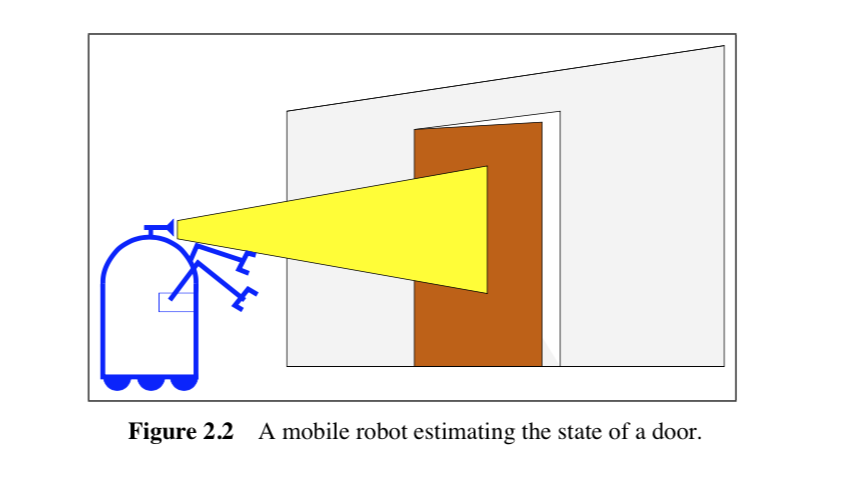# Probabilistic Robotics学习笔记: Bayes Filter 基本概念

## RECURSIVE STATE ESTIMATION

### Introduction

State estimation seeks to recover state variables from the data. Probabilistic state estimation algorithms compute belief distributions over possible world states.

### Bayes Filter

#### Belief Distributions

Bayes Filter 的核心在于对信念分布的操作，所谓信念分布，是在已知传感器数据，动作器动作的情况下对状态$x_t$的一个估计

#### Bayes 的迭代规则

$p(z_i)$为归一化常数，我们在这里仅仅讨论上面二式的物理学意义

$x_t$,$z_t$之间的关系，通过乘因子$p(z_t|x_t)$和归一化后，我们得到了对于x估计的完整值。

#### 一个简单的栗子## “Probabilistic Robotics学习笔记: Bayes Filter 基本概念”的一个回复

1.kx说道：

态0不就跳不出去了吗